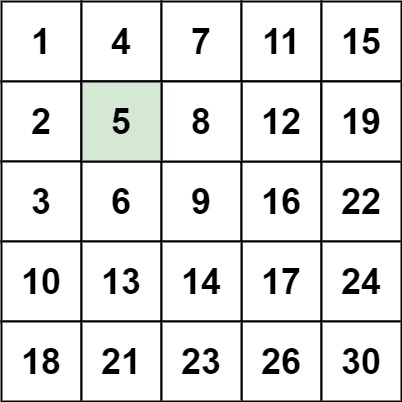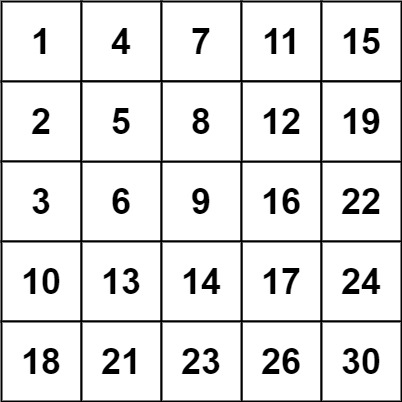0240. 搜索二维矩阵 II

# 0240. 搜索二维矩阵 II#

• 标签：二分查找、分治算法
• 难度：中等

## 题目大意 #

• $m == matrix.length$。
• $n == matrix[i].length$。
• $1 \le n, m \le 300$。
• $-10^9 \le matrix[i][j] \le 10^9$。
• 每行的所有元素从左到右升序排列。
• 每列的所有元素从上到下升序排列。
• $-10^9 \le target \le 10^9$。

• 示例 1：1 2  输入：matrix = [[1,4,7,11,15],[2,5,8,12,19],[3,6,9,16,22],[10,13,14,17,24],[18,21,23,26,30]], target = 5 输出：True 
• 示例 2：1 2  输入：matrix = [[1,4,7,11,15],[2,5,8,12,19],[3,6,9,16,22],[10,13,14,17,24],[18,21,23,26,30]], target = 20 输出：False 

## 解题思路 #

### 思路 1：二分查找 #

1. 迭代对角线元素，假设对角线元素的坐标为 (row, col)。把数组元素按对角线分为右上角部分和左下角部分。
2. 对于当前对角线元素右侧第 row 行、对角线元素下侧第 col 列分别进行二分查找。
1. 如果找到目标，直接返回 True
2. 如果找不到目标，则缩小范围，继续查找。
3. 直到所有对角线元素都遍历完，依旧没找到，则返回 False

### 思路 1：代码 #

  1 2 3 4 5 6 7 8 9 10 11 12 13 14 15 16 17 18 19 20 21 22 23 24 25 26 27 28 29 30 31 32 33 34 35 36 37 38 39 40 41 42 43 44 45 46 47 48 49 50 51 52 53 54 55 56 57 58  class Solution: def diagonalBinarySearch(self, matrix, diagonal, target): left = 0 right = diagonal while left < right: mid = left + (right - left) // 2 if matrix[mid][mid] < target: left = mid + 1 else: right = mid return left def rowBinarySearch(self, matrix, begin, cols, target): left = begin right = cols while left < right: mid = left + (right - left) // 2 if matrix[begin][mid] < target: left = mid + 1 elif matrix[begin][mid] > target: right = mid - 1 else: left = mid break return begin <= left <= cols and matrix[begin][left] == target def colBinarySearch(self, matrix, begin, rows, target): left = begin + 1 right = rows while left < right: mid = left + (right - left) // 2 if matrix[mid][begin] < target: left = mid + 1 elif matrix[mid][begin] > target: right = mid - 1 else: left = mid break return begin <= left <= rows and matrix[left][begin] == target def searchMatrix(self, matrix, target: int) -> bool: rows = len(matrix) if rows == 0: return False cols = len(matrix) if cols == 0: return False min_val = min(rows, cols) index = self.diagonalBinarySearch(matrix, min_val - 1, target) if matrix[index][index] == target: return True for i in range(index + 1): row_search = self.rowBinarySearch(matrix, i, cols - 1, target) col_search = self.colBinarySearch(matrix, i, rows - 1, target) if row_search or col_search: return True return False 

### 思路 1：复杂度分析 #

• 时间复杂度：$O(min(m, n) \times (\log_2 m + \log_2 n))$，其中 $m$ 是矩阵的行数，$n$ 是矩阵的列数。
• 空间复杂度：$O(1)$。